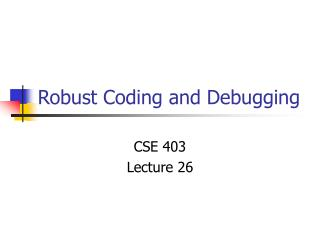DownloadDownload PresentationRobust Coding and Debugging

# Robust Coding and Debugging

Télécharger la présentation## Robust Coding and Debugging

- - - - - - - - - - - - - - - - - - - - - - - - - - - E N D - - - - - - - - - - - - - - - - - - - - - - - - - - -
##### Presentation Transcript

1. Robust Coding and Debugging CSE 403 Lecture 26

3. Don’t do this • #include <stdio.h> char *T="IeJKLMaYQCE]jbZRskc[SldU^V\\X\\|/_<[<:90!\"\$434-./2>]s", K,*F,x,A,*M,*J,r,*g,N,Y,*Q,W,*k,q,D;X(){r [r [r=M[1-(x&1)][*r=W,1],2]=*Q+2,1]=x+1+Y,*g++=((((x& 7) -1)>>1)-1)?*r:r[x>>3],(++x<*r)&&X();}E(){A||X(x=0,g =J ),x=7&(*T>>A*3),J[(x[F]-W-x)^A*7]=Q[x&3]^A*(*M)[2 +( x&1)],g=J+((x[k]-W)^A*7)-A,g=(*M)[*g=M[T+=A ,1 ][x&1],x&1],(A^=1)&&(E(),J+=W);}l(){E(--q&&l () );}B(){*J&&B((D=*J,Q<D&&D<k&&(*g++=1 ), !(D-W&&D-9&&D-10&&D-13)&&(!*r&&(*g++=0) ,* r=1)||64<D&&D<91&&(*r=0,*g++=D-63)||D >= 97&&D<123&&(*r=0,*g++=D-95)||!(D-k[ 3] )&&(*r=0,*g++=12)||D>k&&D<=k[ 1] -1&&(*r=0,*g++=D-47),J++));}j( ){ putchar(A);}b(){(j(A=(*K)[D* W+ r*Y+x]),++x<Y)&&b();}t () {(j((b(D=q[g],x=0),A=W) ), ++q<(*(r+1)<Y?*(r+1): Y) )&&t();}R(){(A=(t( q= 0),'\n'),j(),++r [2 ]<N)&&R();}O() {( j((r=0,R( )) ),r-=q) && O(g-=-q) ;} C(){( J= gets (K ))&&C((B(g=K),*r=!(!*r&&(*g++=0)),(*r)[r]=g-K,g=K[2 ],r[ 1]&& O()) );;} main (){C ((l( (J=( A=0) [K], A[M] =(F= (k=( M[!A ]=(Q =T+( q=(Y =(W= 32)- (N=4 )))) +N)+ 2)+7 )+7) ),Y= N<<( *r=! -A)) );;}

4. Assertions • Don’t use assertions to check unusual conditions • You need explicit error code for this • Only use them to ensure that illegal conditions are avoided

5. Memory • The memcpy examples are from Writing Solid Code: Microsoft's Techniques for Developing Bug-Free C Programs • Although the book is general, lots of the guidelines focus on memory issues • Marking freed memory • Not accessing freed memory • Dealing with details of realloc • These are real issues, but appear less frequently in other languages

6. Writing solid code • Shred your garbage • Force early failure, increase determinism • Why 0xA3? void FreeMemory(void *pv){ Assert(pv != NULL); memset(pv, 0xA3, sizeofBlock(pv); free(pv);}

7. Should debug code be left in shipped version • Pro: • Debug code useful for maintenance • Removing debug code change behavior • Bugs in release but not debug versions • Con: • Efficiency issues • Different behavior for debug vs. release • Early fail vs. recover

8. Step through your code • Maguire • Step through new code in the debugger the first time it is used • Add code, set break points, run debugger • Add code, run tests, if failure, run debugger • Knuth • Developed tool to print out first two executions of every line of code

9. Candy machine interfaces • Error prone return values or arguments • Classic bad example, getchar() returns an int! • Alternate approach • bool fGetChar(char pch); • Many bugs with malloc returning NULL char c; c = getchar(); If (c == EOF) …

10. Another coding quiz char tolower(char ch){ }

11. Handling out of range inputs • Ignore • Return error code • Assert • Redefine the function to do something reasonable • Write functions that, given valid inputs, cannot fail

12. Debugging • What are the key steps in debugging a program?

13. Kernigan and Pike's debugging wisdom • Look for common patterns • Common bugs have distinct signatures • int n; scanf("%d", n); • Examine most recent change • Don't make the same mistake twice • Debug it now, not later • Get a stack trace • Read before typing

14. K & P, II • Explain your code to someone else • Make the bug reproducible • Divide and conquer • Find simplest failing case • Display output to localize your search • Debugging with printf() • Write self checking code • Keep records

15. My favorite bugs (and stupidities) • BI280 Business Basic Interpreter written in C for CP/M • Sporadic failure of parsing • Only happened when Basic program was changed (after being loaded) • Parsing done by interpreter, each time a line was executed • Adding printf's to code also changed behavior

16. The Bug • Uninitialized variable • Variable used by the parser to hold a character that would be either a binary operator or end of line • Parsing algorithm looked at last character and tested if it was a binary operator to continue parsing

17. Don't do this • Can cover up very bad things • Violates K&P: Debug it now, not later try { doSomething(); } catch (Exception e){ }

18. Apocryphal (but still a good story) • A program which fails only in the month of September

19. Apocryphal (but still a good story) • A program which fails only in the month of September char monthName; strcpy(monthName, "September");

20. ConferenceXP • Video conferencing system would run (after initial install) for about an hour and then fail • System would not work at all at this point • In a week it would start working again (for an hour) • Repeated recovery every week

21. Solution • Install process (erroneously) set event log to "overwrite events older than 7 days • Application was very verbose in logging • Failure when log was full